### Sample Problem

We know that the vertices (-1, 3), (3, 5), (5, 1) form a right triangle because:

#### Solution

Calculate the lengths of the sides of the triangle in order to confirm that according to the Pythagorean theorem, the sum of the lengths’ squared is equal to the hypotenuse squared (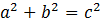).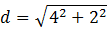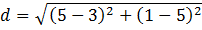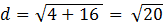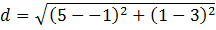Therefore, the answer is (C).